﻿ New Chart Types for Smarter Data Visualization in Reporting for WinForms# New Chart Types for Smarter Data Visualization in Reporting for WinForms

​​There are various situations where you need smarter data visualization capabilities to interpret data and make quick decisions. ​Thw 2015 v2 release added these capabilities for you by introducing new charts in Reports for WinForms.​

## Step Chart

​​​​​​For interpreting data that changes ​occasionally, but remains constant for a period of time, Step Chart is the solution to your problem. The following example demon​strates the usage of Step Chart in C1ReportDesigner. The following Table shows Home Loan Interest Rates from Jan 2015 - Apr 2016 period: ​​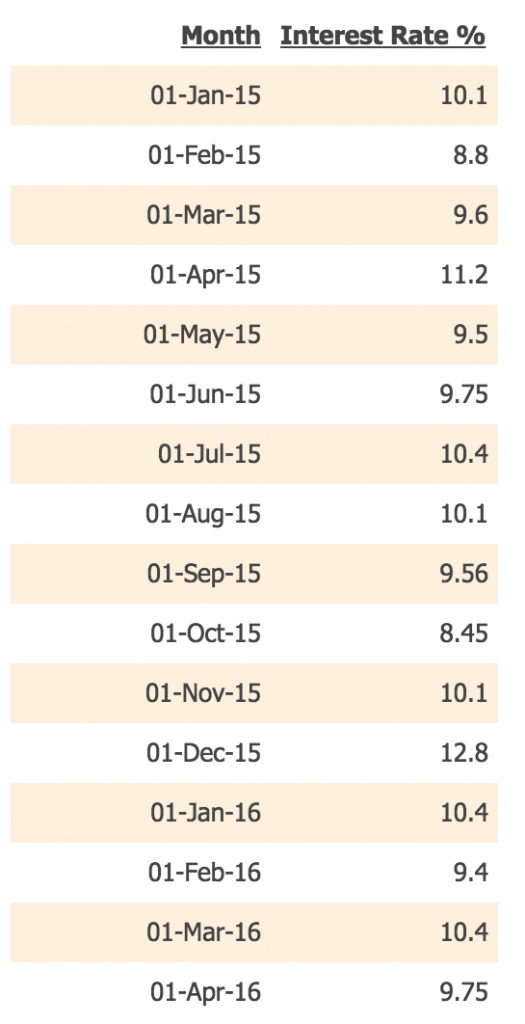This data shows that interest rates change every month, but remains constant during the month period. It is useful to visualize such data using Step Chart. In order to plot this data, follow the following steps: Note:Use C1ReportDesigner to create a report containing Step Chart.

1. Open C1ReportDesginer
2. Create New Report and bind it to StepPoints Table in Data.mdb (see New Charts link in Report section)
3. Drop Chart Field on the Designer.
4. Set ChartType as Step.
5. Set DataX as Month.
6. Set DataY as [Interest Rate %].
7. Set DataColor as DarkSalmon.
8. Set LabelCompass as NorthEast.

​And you're done! Here's your Step Chart: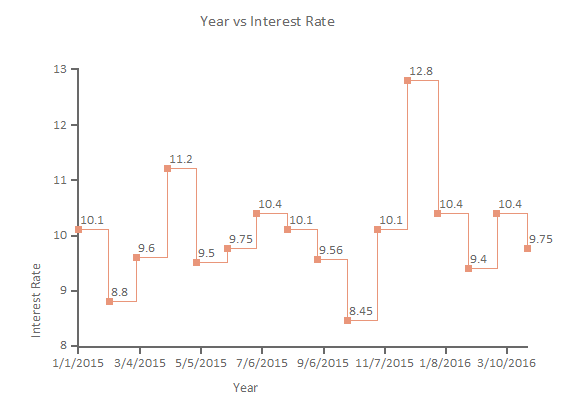The Chart shows Step-wise increase/decrease of Interest Rates during Jan 2015-Apr-2016.

​When data is in the form of a two-dimensional chart with three or more quantitative variables, it is more helpful to plot and view such data using a Radar Chart. Consider an example where you want to evaluate multiple Mobile brands on features like battery, memory, UI, camera & brand. These features are rated on a scale of 1-5, so that the data looks like this: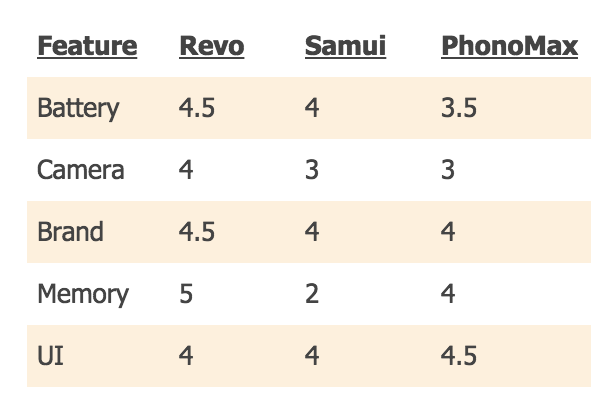Now, a simple visualization can be done by using a Column Chart, but when the number of features becomes large, the Column Chart might get cluttered. In such cases, Radar Chart can plot data in a neater way using the following steps:

1. Open C1ReportDesigner.
2. Create New Report and bind it with MobileData table in Data.mdb (see New Charts link in Report section)
3. Drop Chart Field on the Designer.
5. Set DataX as 'Feature'.
6. Set DataY as 'Revo; Samui; PhonoMax'.
7. Set Palette as 'Teal;Tomato;SandyBrown'.
8. Set Radar->FlatGridLines False for Circular plotting, and True for Flat Lines for Y coordinate gridlines.

And your Radar chart is ready. For FlatGridLines=False, you'l obtain the following chart: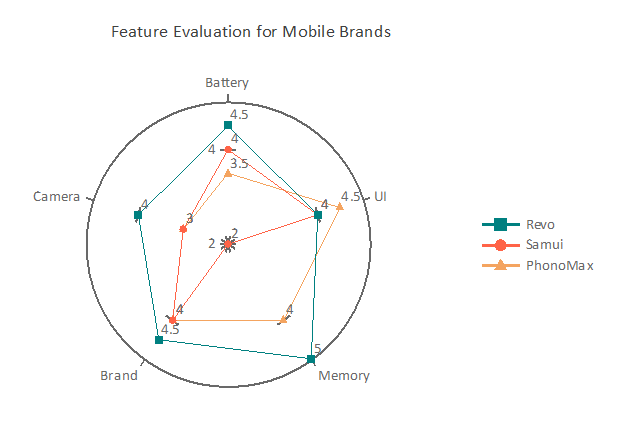FlatGridLines=True results in following chart -

## Polar Chart

For certain phenomena, that is characterized by direction and distance from a fixed point, the corresponding data can be plotted on a Polar Chart. Polar Charts are also useful for intuitive visualization of multivariate data. ​​ The following Table shows the Speed of a boat vs different Angles of Boat Direction for Wind Speed (90 degrees)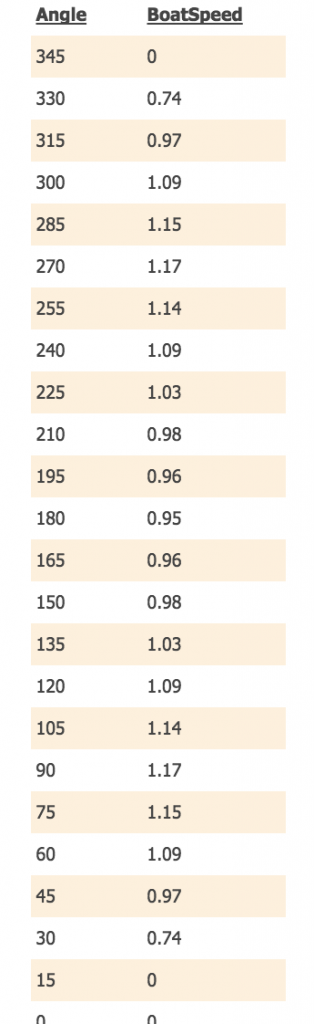The data demonstrates that if Wind Direction is 90 degrees, the speed of the boat changes w.r.t different angles of Boat Direction. It is observed that if the boat moves 90-105 degrees or 255-285 degrees, the boat speed is maximum. ​In order to plot this data, use following steps:

1. Open C1ReportDesigner.
2. Create New Report and bind it with PolarSample table in Data.mdb (attached below)​.
3. Drop Chart Field on the Designer.
4. Set ChartType as Polar.
5. Set DataX as 'Angle'.
6. Set DataY as 'BoatSpeed'.
7. Set Polar->Degrees to True (in order to plot data in degrees)
8. Set StartAngle to 90 (or set it as per your choice)
9. Set DataColor as Teal

Following is a snapshot of a Polar Chart you have accomplished.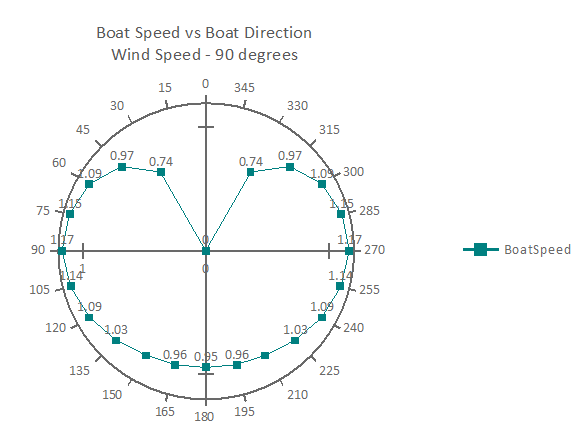## Histogram Chart

When your data falls in specific intervals, it is more beneficial to analyze it using a Histogram Chart over a Bar Chart since it can organize a group of data points into user-specified ranges. Here is how Histogram Chart in C1Report works:

It takes a collection of raw data values and plots the frequency distribution. The data is processed in the following way -

1) Gets raw data from Y values.

2) Analyzes the raw data with the interval setting. The result is the distribution (count) of each interval.

3) Draws the Histogram with Axis-X with intervals and Axis-Y with frequency distribution (count).

The following is a simple example of frequency distribution for Height (in inches) of Students.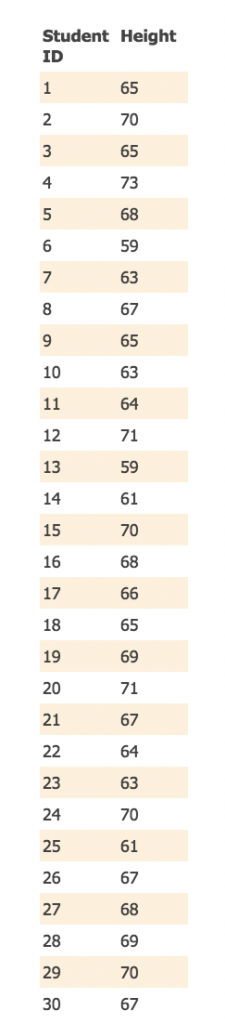Below are the steps to plot this data in a Histogram Chart

Note: This method uses IntervalCreationMethod as SemiAutomatic. In a SemiAutomatic method, the upper and lower limits of the intervals are specified together with the number of intervals. The Interval boundaries are calculated uniformly. The IntervalStart, IntervalWidth, and IntervalNumber properties are available when you select the SemiAutomatic method. The IntervalStart property gets or sets the numeric value of the beginning of the first interval.

1. Open C1ReportDesigner.
2. Create New Report and bind it with StudentHeight table in Data.mdb (attached below)​.
3. Drop Chart Field on the Designer.
4. Set ChartType as Histogram.
5. Set DataX as ID (which is actually ignored)
6. Set DataY as Height.
7. Expand 'Histogram' Property.
8. Set IntervalCreationMethod as 'SemiAutomatic'.
9. Set IntervalNumber as 11 (because the Heights range from about 55-71 inches, so dividing with intervals of width 2, atleast 11 intervals are needed to show the count)
10. Set IntervalStart as 54 (in order to accomodate 11 intervals and Height range till 74 inches)
11. Set IntervalWidth as 2 (so that Heights from 54-71 can be accomodated on the X-Axis)
12. Expand 'NormalDisplay'.
13. Set FillStyle->Color1 as 'Transparent'.
14. Set ForegroundDisplay as 'True'.
15. Set 'Visible' as True.

The resulting Histogram Chart looks like this -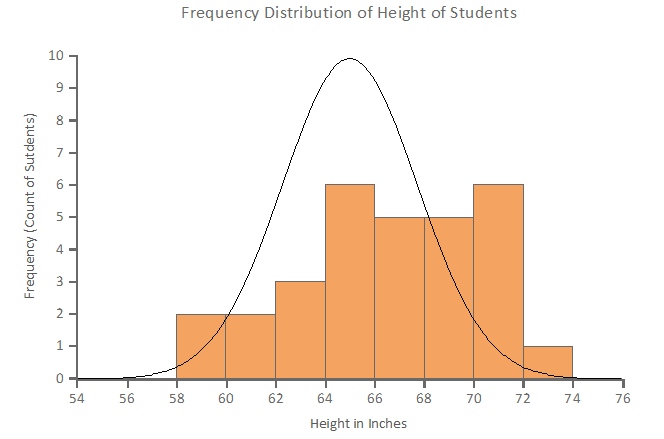The chart depicts that there are 2 students for Height Interval 58-60 inches, 2 students in Interval 60-62 inches and so on. The Histogram calculates the count of students internally for the specified IntervalNumber (i.e. 11).

## Important Note